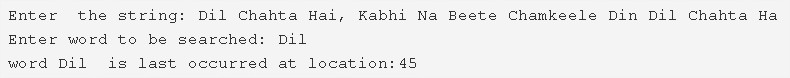# C Program To Find Last Occurrence Of A Word In A String | C Programs

C program to find the last occurrence of a word in a given string – In this article, we will summarise in on the various techniques to find the last occurrence of a word in a given string in C programming.

Suitable examples and sample programs have also been added so that you can understand the whole thing very clearly. The compiler has also been added with which you can execute it yourself.

The methods used in this piece are as follows:

• Using Standard Model
• Using Function
• Using Recursion

A string is nothing but an array of characters. The value of a string is determined by the terminating character. Its value is considered to be 0.As you can see in the mentioned example, firstly, you need to enter the particular string.

The string entered here is “Dil Chahta Hai, Kabhi Na Beete Chamkeele Din Dil Chahta Hai”.

Afterwards, you need to specify the word that you need to search.

In this case, the word is “Dil”.

Hence, it is seen that the word “Dil” last occurs at location 45.

Thus, doing the same in C programming is as follows:

## Using Standard Method

1. Read the entered string and store the string into the variable ‘s’ using gets(s) function.

2) Read the entered word to be searched and store the word in the variable w using gets(w) function.

3) Using first for loop store the locations of white space in the integer string a[].

4) Initialize j=length of the string.

5) The for loop iterates through white spaces of the string in reverse order with the structure for(k–;k>=0;k–)

a) Initialize n=length of the string-index of the white space a[k]-1.

b) If n is equal to the length of the word

Then initialize t=0 and compare the first element of the word with the element of the string with the index a[k]+l+1. If matches then increase the t value. Repeat this until the last element of the word.

c) If t=length of the word then initialize found=1 and break the for loop. Otherwise initialize  j=a[k].

6) If found=1 then print the location of the last occurrence of the word in the string. Otherwise, print the word has not occurred in the string.

Output:

### Using Function

1. The main() calls the check(char *s, char *w) function to find the location of the last occurrence of the word in the string.

2) The check function

a) It stores the locations of the white space of the string in the integer string a[].

b) Initialize j=length of the string.

c) The for loop iterates through the white spaces of the string in reverse order with the structure for(k–;k>=0;k–).

n=length of the string a[k]-1.

if n=length of the word then t=0. Compare the elements of the word w with the element of the string at the index a[k]+l+1. If matches then increase t value. Repeat until the last element of the word.

if t=1 then initialize found=1 and break the for loop.Otherwise initialize j=a[k].

3) If found=1 then it returns the location of the last occurrence of the word.i.e a[k]+1.Otherwise, return -1.

4) If the function return value is >0 then the main() prints the location of the last occurrence of the word in the string value. Otherwise, print the word has not occurred in the string.

Output:
x

## C Program To Print Number Of Days In A Month | Java Tutoring

C program to input the month number and print the number of days in that ...